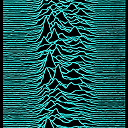Score:2Crypto

# How to check whether function provides full diffusion or not?In "The Skein Hash Function Family" paper authors wrote:

The MIX/permute structure has been designed to provide full diffusion in 9 rounds for Threefish-256, 10 rounds for Threefish-512, and 11 rounds for Threefish-1024.

How they know it? Is there some rule of designing such structures, which can quarantee some level of diffusion and it was used there (then they could estimate the number of rounds needed directly)? Or they measure diffusion experimentally?

By the way they further write:

We designed Skein to maximize diffusion at every level, and have defined the number of rounds to be high enough to allow for many full diffusions. Each input bit position affects every output bit position in 10 rounds for Skein-512 (9 rounds for Skein-256 and 11 rounds for Skein-1024), so the algorithm is specified with 7–8 full diffusions. By comparison, AES-128 and Twofish have only 5 full diffusions.

So maybe this is how they figured it out. But without precise analysis this still not clear to me, especially because mix function in Threefish is so simple and there is not much more going on there, than that mixing.It's easy to determine what changing an input bit can and cannot change at later stages. That can be either on a drawing representing a few rounds that one colors according to if there can be an influence or not; or on instrumented software. I recommend it as an exercise.
Score:3CryptoDiffusion is the same as mixing.

Read section 8.3 as they suggest. A lot of it for this simple structure depends on the choice of the rotation constants, and those were experimentally optimized using an evolutionary algorithm.

In the case of AES, the use of MDS codes means that 5 of 8 bytes are always "active" (the minimum weight/distance of the MDS code with parameters $$[n,k]=[8,4]$$ is $$d=n-k+1=5.$$) This means that you get diffusions (maximum possible under linear mixing due to the MDS bound on $$d$$) of the type

• 1 nonzero byte (of the $$(a_{i,j})_{i=1,\ldots,4}$$) going to at least 4 nonzero bytes (of the $$(b_{i,j})_{i=1,\ldots,4}$$)
• 2 nonzero bytes (of the $$(a_{i,j})_{i=1,\ldots,4}$$) going to at least 3 nonzero bytes (of the $$(b_{i,j})_{i=1,\ldots,4}$$)
• 3 nonzero bytes (of the $$(a_{i,j})_{i=1,\ldots,4}$$) going to at least 2 nonzero bytes (of the $$(b_{i,j})_{i=1,\ldots,4}$$)
• 4 nonzero bytes (of the $$(a_{i,j})_{i=1,\ldots,4}$$) going to at least 1 nonzero bytes (of the $$(b_{i,j})_{i=1,\ldots,4}$$)

in each of the columns in MixColumns.To explain further, the Singleton Bound Wikipedia states that for any code over a symbol alphabet with $$q$$ elements, the minimum distance (and thus for a linear code the minimum weight, i.e., the number of nonzero components) satisfies $$d\leq n-k+1$$ where $$n$$ is the length of the codewords, $$k$$ is the dimension of the code. MDS is maximum distance separable, i.e., optimal, i.e., the largest possible minimum distance for given $$n,k$$ parameters.

We have $$q=2^8,$$ here since we consider each byte as a code symbol. Since the column of $$b'$$s is obtained by multiplying the column of $$a$$'s with an MDS matrix the two columns form codewords in this code. Thus $$d=n-k+1=5$$ thus at least 5 of the bytes in the $$b$$'s column and the corresponding $$a$$'s column are guaranteed to be nonzero.

This is an algebraic design. In the Skein spec they use the somewhat strange expression 5 full diffusions for AES, presumably related to this value of 5 nonzero bytes.

See here for more details on the AES mixing design.I sit in a Tesla and translated this thread with Ai: